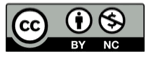# 如何用代码模拟自由落体

2019-05-30## 模型

$T$ 为相邻两帧的间隔时间。上式中 $g_0$, $T$ 都为常量，我们可以合并为一个新的常量 $g_1$，这样 $v(n)$ 构成了一个离散序列:

$$v(n)=g_1n$$或者$$v(n)=v(n-1)+g_1 \tag{1}$$

## 实现

var y=0, n=0, g=1
while(小球未落地)
y+=(n++)*g


（点击动画重新播放）

## 落地反弹

var y=0, v=0, g=1
while(小球未落地)
v+=g
y+=v


var y=0, v=0, g=1
while(true)
if(小球触碰地面){
v=-v
}
v+=g
y+=v


## 考虑损耗

var y=0, v=0, g=1, f=0.6
while(true)
if(小球触碰地面){
v=-f*v
}
v+=g
y+=v


（点击动画重新播放）

（完）

## 精彩评论